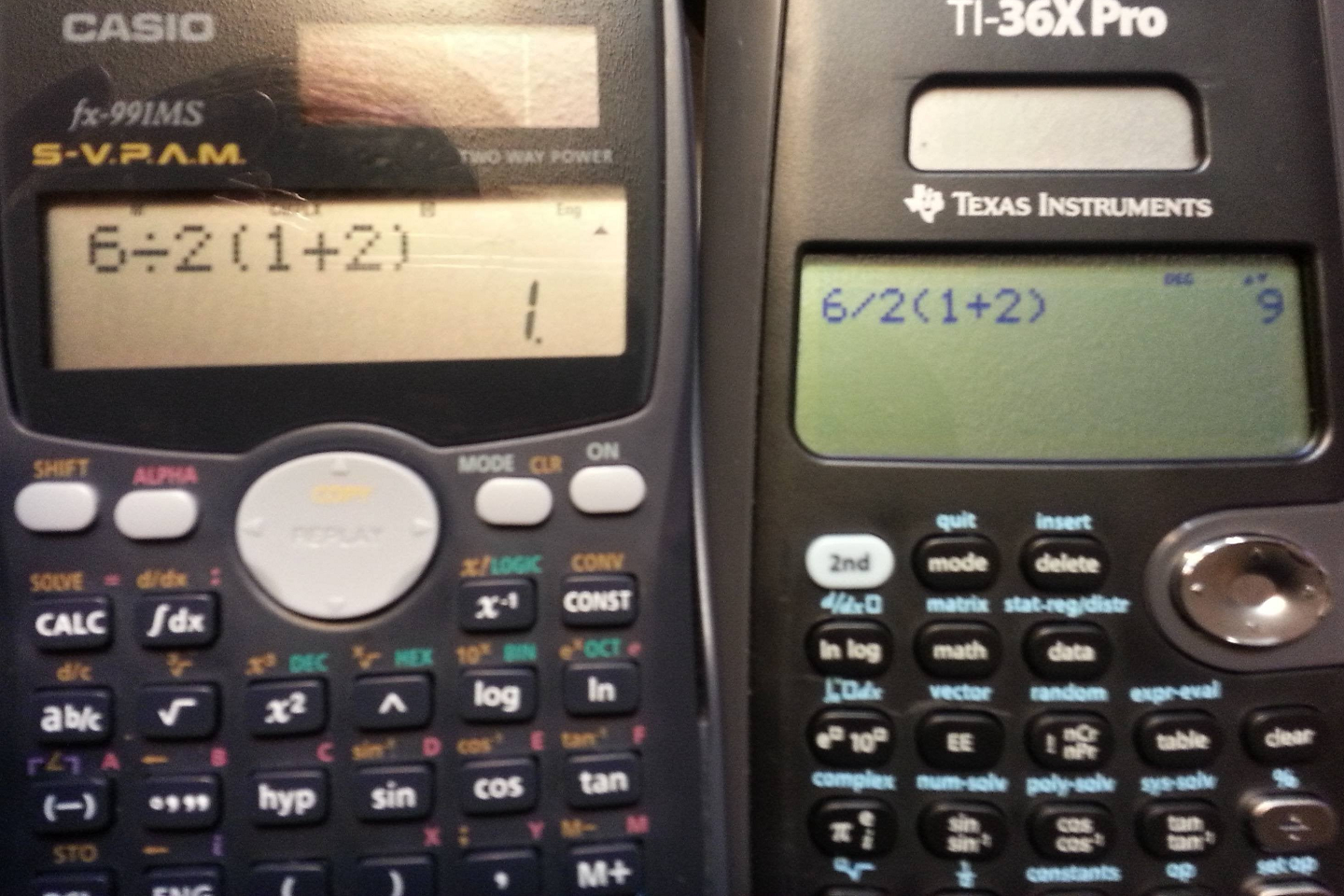# The Implications of Being Implicit

July 17, 2014
Mathematics

In my previous post, I presented three "tricky" (read: "inane") math problems from the Internet, the last of which was the following:

I promised I'd come back to this one because it merits special discussion.  Now it's time to do exactly that, as this one (as well as its many variations) has piqued my ire every time I've seen it.

Most people who have had at least a basic prealgebra class tend to agree that the $$1+2$$ in parentheses should be evaluated first, reducing the problem to $6\div 2(3).$
After that the battlefield gets bloody.

Do we do the multiplication first, or do we do the division first?

Some people say the multiplication needs to come first, valiantly shouting "PEMDAS" as their battle cry, arguing that since "M" comes before "D", the answer is $$1$$.

Those who have more experience with order of operations and don't just rely on a silly (and wrong) mnemonic say that the division comes first, since multiplication and division are really the same under the hood - after all, division is equivalent to multiplication by the reciprocal - and by convention* are performed from left to right in the order they appear.  For these more seasoned warriors, the answer is "obviously" $$9$$.

Those in the latter camp definitely are applying better mathematical reasoning than those in the former.  But do they have "the" correct answer?  If you're like me, though you know that multiplication and division are supposed to happen in order from left to right, there's just something about that $$2(3)$$ that catches your eye, that makes you feel like for some reason it "should" come first.

And that's why we need to talk about implicit (or implied) multiplication.

When we first learn multiplication, we write it with a cross ($$\times$$).  But once variables like $$x$$ start to come into play, we have to find new, less confusing ways to write multiplication.  So instead of writing $$a\times b$$, we have a few options.

• We can use a dot: $a\cdot b$
• We can use parentheses: $a(b)$
• Or as long as both factors aren't numerals, we can just concatenate (attach) them:  $ab$

There's actually a subtle difference between multiplication with a cross or a dot and multiplication with parentheses or concatentation.  The former two are called explicit multiplication, because we've explicitly indicated our operation using a symbol.  The latter two are called implicit multiplication - we know the intended operation is multiplication because no explicit symbol was provided.

Why does this matter?  As it turns out, in some conventions, implicit multiplication may actually take precedence over explicit multiplication and therefore division!  For example, the "Style and Notation Guide" for Physical Review, an American scientific journal, specifies that implicit multiplication should come before division when submitting manuscripts**.  Of course there are other conventions in which this is not the case, but the point to understand here is that multiple conventions do exist.

What's more, we can't even turn to our trusty calculators to tell us which way is "the" correct way, because different calculators may follow different conventions!

(Try this on your own calculator and see which convention it uses!)

I'd like to posit one more reason that the $$2(3)$$ may feel like it "should" come first.  One unfortunate side effect of trying to use existing punctuation when possible to represent mathematics is that certain symbols become overloaded - the same symbols can represent different things.  In this case, the notation $$2(3)$$ for multiplication bears a very strong resemblance to the notation $$f(3)$$ for function evaluation!  If the question were $6\div f(1+2),$ even though we have no idea what function $$f$$ is, there's no question that it would be evaluated before the division took place!  This may be a possible reason that the implicit-trumps-explicit convention is followed in some circles.

The inevitable conclusion is that there is no single correct answer - it all depends on what convention you're using.  At this point you may be ready to throw your hands up in despair.  But there is hope.  The best way to solve this kind of problem is ... you guessed it ... to use better notation in the first place!  (I mean who uses the obelus ($$\div$$) anymore past 5th grade anyway?)

• If you mean for multiplication to be done first, then say so!  $\frac{6}{2(1+2)}=1$
• If you mean for division to be done first, then say so!  $\frac{6}{2}(1+2)=9$

And if you're using a calculator, it never hurts to have too many parentheses.***

It all comes back to the point of the previous article, which I will make explicit one more time:  Math isn't about symbols.  Math is about ideas.  If your symbols don't unambiguously convey your ideas, then use better symbols.

* The left-to-right convention is probably so because those who established the convention spoke the sorts of European languages for which $$6\div 2$$ would be vocalized in that order - not always the case if you've ever heard how fractions are read out loud in Japanese or Korean!

** The guide may seem like it's claiming that all multiplication should come before division, but this is because they don't use explicit multiplication at all except in specific contexts such as indicating dimensions and performing operations on vectors.

*** If you have a newer calculator, you may have a nifty fraction template that you can use to clear up confusion even further!  If you're using a TI-84+, and you've got the newest operating system on your calculator, try hitting [ALPHA] and then [Y=].  If you see a little menu come up, choose "n/d" and voilà - you can now do fractions without getting lost in a sea of parentheses!